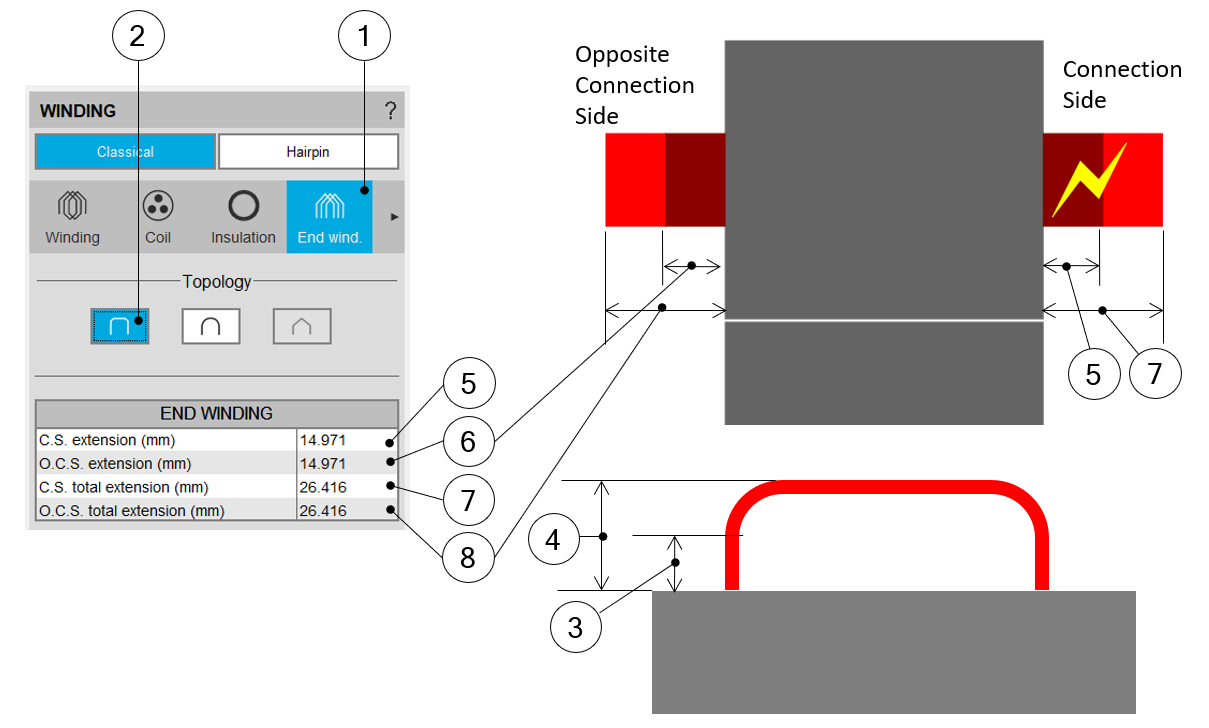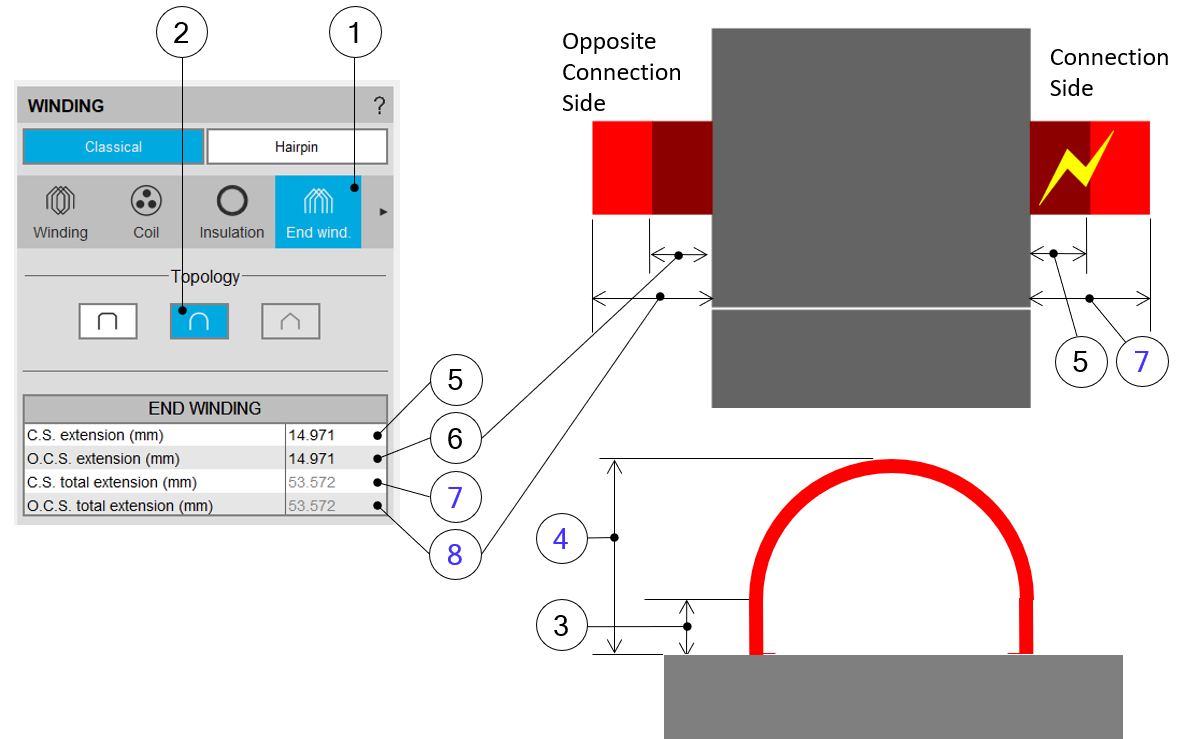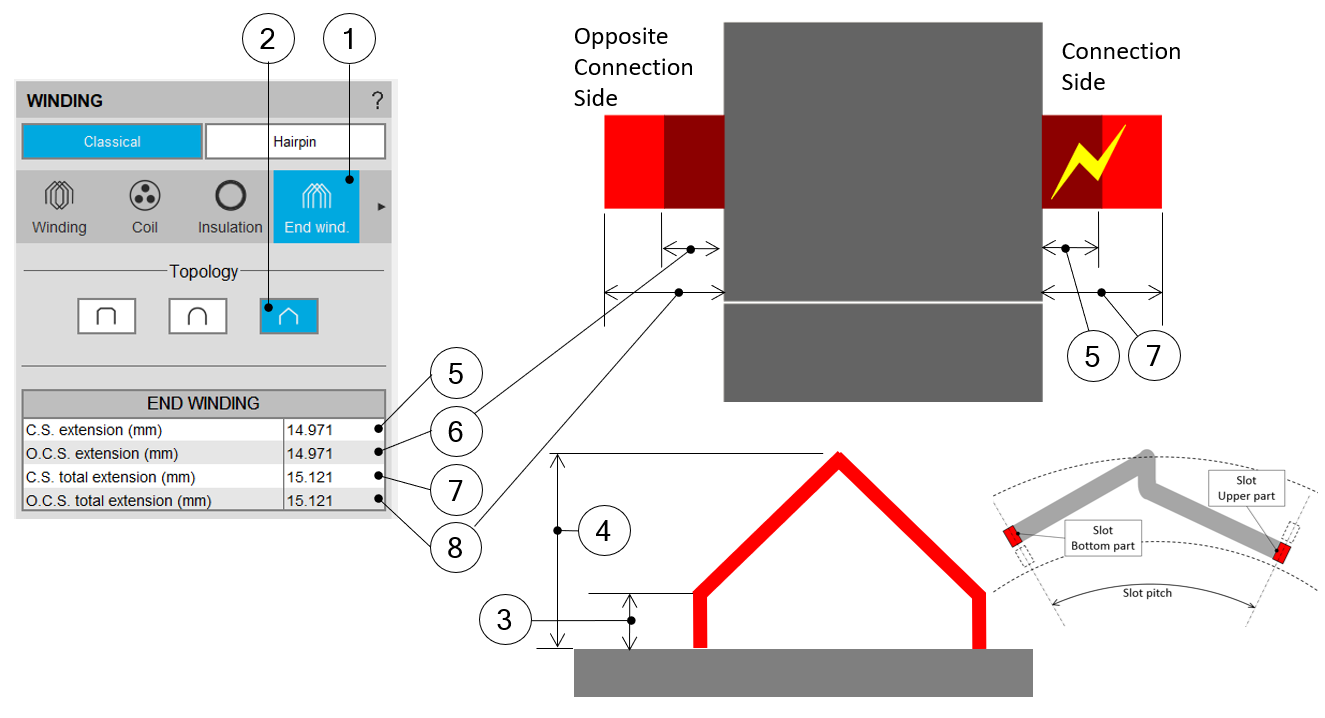# End windings design

## 1. Overview - definitions

This part characterizes the end-winding and the resulting conductor dimensions.

For additional information refer to the sections dedicated to the coil and conductor settings and End-winding topology.

 Label Symbol Tooltip, note, formula End-winding topology * End-winding topology: U-shape, C-shape or Y-shape. C.S. total extension * Connection side total extension. C.S. straight extension * Connection side straight extension Axial overall length * Axial overall length. Length between the two extremities of the winding i.e. between connection side and opposite connection side. O.C.S. total extension * Opposite connection side total extension. O.C.S. straight extension * Opposite connection side straight extension. Total conductor length * Total conductor length. Mean turn length * Mean turn length. Coil connection length * Additional length corresponding to the connections between coils.

## 2. End-winding topology – U-Shape

Topology available for all the 3 winding architecturesBuilding the winding – End-winding topology and dimensionsCase of the U-shape End-winding 1 Selection of the END-WINDING tab. 2 Selection of the U-Shape end-winding case. 3 Straight extension of the U-Shape end-winding topology = User input parameter. 4 Total extension of the U-Shape end-winding topology = User input parameter. 5 Definition of the connection side straight extension (ref. 3). 6 Definition of the opposite connection straight extension (ref. 3). 7 Definition of the connection side total extension (ref. 4). 8 Definition of the opposite connection side total extension (ref. 4).

## 3. End-winding topology – C shape

Topologies available for all winding architectureBuilding the winding – End-winding topology and dimensionsCase of the C-shape End-winding 1 Selection of the END-WINDING tab. 2 Selection of the C-Shape end-winding topology. 3 Straight extension of the C-Shape end-winding topology = User input parameter. 4 Total extension of the C-Shape end-winding topology = Computation result deduced from topology characteristics. 5 Definition of the connection side straight extension = User input (ref. 3). 6 Definition of the opposite connection straight extension = User input (ref. 3). 7 Definition of the connection side total extension = Deduced result (ref. 4). 8 Definition of the opposite connection side total extension = Deduced result (ref. 4).

## 4. End-winding topology – Y shape

This topology is available only with two layers and superimposed coil layout.Building the winding – End-winding topology and dimensionsCase of the Y-shape End-winding 1 Selection of the END-WINDING tab. 2 Selection of the Y-Shape end-winding case. 3 Straight extension of the Y-Shape end-winding topology = User input parameter. 4 Total extension of the Y-Shape end-winding topology = User input parameter. 5 Definition of the connection side straight extension (ref. 3). 6 Definition of the opposite connection straight extension (ref. 3). 7 Definition of the connection side total extension (ref. 4). 8 Definition of the opposite connection side total extension (ref. 4).